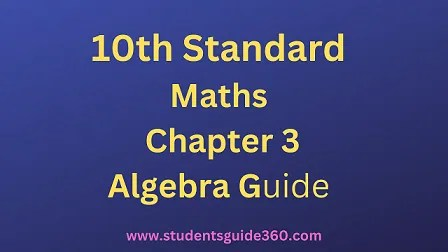# 10th Maths Guide Chapter 3. Algebra Exercise 3.10

10th Standard Maths Chapter 3 Algebra Samacheer Kalvi Guide Exercise 3.10 Book Back Answers Solutions. TN 10th SSLC Samacheer Kalvi Guide. 10th All Subject Guide – Click Here. Class 1 to 12 All Subject Book Back Answers – Click Here## 10th Maths Guide Chapter 3. Algebra Exercise 3.10

### 1. Solve the following quadratic equations by factorization method

• (i) 4×2 – 7x – 2 = 0
• (ii) 3(p2 – 6) = p(p + 5)
• (iii) √a(a−7)=3√2
• (iv) √2×2+7x+5√2=0
• (v) 2×2 – x + 1/8 = 0

Solution:(ii) 3(p2 – 6) = p(p + 5)
3p2 – 18 = p2 + 5p ⇒ 392 – 5p – 18 = 0
⇒ 2p2 – 5p – 18 = 0
⇒ (2p – 9) (p + 2) = 0 ⇒ p = 92, -2

(iii) a(a−7)−−−−−−−√=32–√
Squaring on both sides
a(a – 7) = 9 × 2
a2 – 7a – 18 = 0
a2 – 9a + 2a – 18 = 0
a(a – 9) + 2(a – 9) = 0
(a – 9) (a + 2) = 0
⇒ a = 9, a = -2(v) 2×2 – x + 18 = 0
16×2 – 8x + 1 = 0
16×2 – 4x – 4x + 1 = 0
4x(4x – 1) – 1(4x – 1) = 0
(4x – 1) (4x – 1) = 0
⇒ x = 14,14